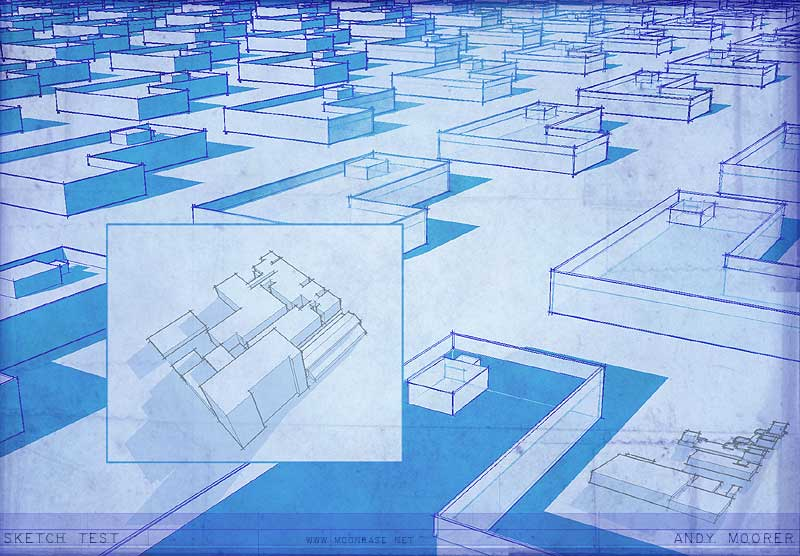# Matic3D

Members

3

0 Neutral

• Rank
Peon

## Personal Information

• Name
Andy
• Location
USA
1. It's not trivial, as the number of nodes he seems to have used suggests. But play with the carve node, to reveal wireframes and attribute transfer for the "flipping polygons" reveal and if you can work up an approximate low-res prototype that will give you room to ask more specific questions. There's no tutorial for the specific effect that I'm aware of, and this is an effect that took top professionals serious effort (and \$\$) to make... so you have to take small bites at a time to replicate it. (And you might want to delete the multiple questions asking the same thing, I'm new here but suspect that just will piss people off... ) Cheers.
2. Lol yes much simpler... I figured it out around the time you posted and my message has been edited for reference. Thanks Tomas! I'm finding optransform and cracktransform to be pretty handy. Now in my 9'th week of houdini after moving over from ICE and liking it more and more, cheers.
3. For the record, there is a way to do this, but it's fugly... since coming up with this I have moved to using SpencerL's approach of using an objectmerge Sop instead... but in case someone really needs it, here it is. The full wrangle for making a matrix out of this should be straightforward but for reference it is on http://andy.moonbase.net. Cheers. // Create vectors to fill a matrix using the second node input's transform (ie a null) vector translate = set(`chs(opinputpath(".", 1)+"/tx")`, `chs(opinputpath(".", 1)+"/ty")`, `chs(opinputpath(".", 1)+"/tz")`); vector rotate = set(`chs(opinputpath(".", 1)+"/rx")`, `chs(opinputpath(".", 1)+"/ry")`, `chs(opinputpath(".", 1)+"/rz")`); vector scale = set(`chs(opinputpath(".", 1)+"/sizex")`, `chs(opinputpath(".", 1)+"/sizey")`, `chs(opinputpath(".", 1)+"/sizez")`); But there's a much cleaner way, too. Get a matrix for your object (a camera or null usually) via optransform and then use the cracktransform function if you need some portion of the matrix, in this example I extract out the position. (The result is a simple way for getting the position of the camera in vex, which is a subject on a bunch of threads here.) vector @cdir; matrix camMatrix = optransform(chs("camera")); //get a matrix with the camera's transforms. @cdir = cracktransform(0, 0, 0, {0,0,0}, camMatrix); //extract out the camera position as a vector
×
• Donations